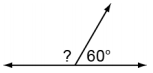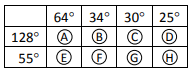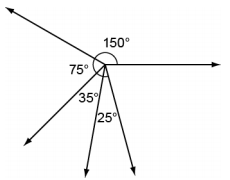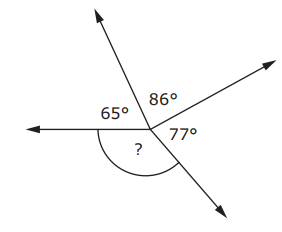# MAFS.4.MD.3.7

Recognize angle measure as additive. When an angle is decomposed into non-overlapping parts, the angle measure of the whole is the sum of the angle measures of the parts. Solve addition and subtraction problems to find unknown angles on a diagram in real world and mathematical problems, e.g., by using an equation with a symbol for the unknown angle measure.

General Information
Subject Area: Mathematics
Domain-Subdomain: Measurement and Data
Cluster: Level 2: Basic Application of Skills & Concepts
Cluster: Geometric measurement: understand concepts of angle and measure angles. (Additional Cluster) -

Clusters should not be sorted from Major to Supporting and then taught in that order. To do so would strip the coherence of the mathematical ideas and miss the opportunity to enhance the major work of the grade with the supporting clusters.

Date of Last Rating: 02/14
Status: State Board Approved
Assessed: Yes
Test Item Specifications

• Assessment Limits :
Whole number degree measures, sums, and differences may only be within 0° and 360°.
• Calculator :

No

• Context :

Allowable

Sample Test Items (4)
• Test Item #: Sample Item 1
• Question:

What is them measure of the unknown angle?• Difficulty: N/A
• Type: MC: Multiple Choice

• Test Item #: Sample Item 2
• Question:

Kyle is adding angles to create other angles.

Fill in the circles to select the angles Kyle can use to create a 128º angle.

Fill in the circles to select the angles that Kyle can use to create a 55º angle.• Difficulty: N/A
• Type: MI: Matching Item

• Test Item #: Sample Item 3
• Question:

A diagram is shown.What is the sum of all the angles that are labeled?

• Difficulty: N/A
• Type: EE: Equation Editor

• Test Item #: Sample Item 4
• Question:

What is the measure, in degrees (º), of the missing angle?• Difficulty: N/A
• Type: EE: Equation Editor

## Related Courses

This benchmark is part of these courses.
5012060: Mathematics - Grade Four (Specifically in versions: 2014 - 2015, 2015 - 2022 (current), 2022 and beyond)
7712050: Access Mathematics Grade 4 (Specifically in versions: 2014 - 2015, 2015 - 2018, 2018 - 2022 (current), 2022 and beyond)
5020110: STEM Lab Grade 4 (Specifically in versions: 2016 - 2022 (current), 2022 and beyond)
5012055: Grade 3 Accelerated Mathematics (Specifically in versions: 2019 - 2022 (current), 2022 and beyond)
5012015: Foundational Skills in Mathematics 3-5 (Specifically in versions: 2019 - 2022 (current), 2022 and beyond)

## Related Access Points

Alternate version of this benchmark for students with significant cognitive disabilities.
MAFS.4.MD.3.AP.7a: Find sums of angles that show a ray (adjacent angles).

## Related Resources

Vetted resources educators can use to teach the concepts and skills in this benchmark.

## Assessments

Sample 3 - Fourth Grade Math State Interim Assessment:

This is a State Interim Assessment for fourth grade.

Type: Assessment

Sample 2 - Fourth Grade Math State Interim Assessment:

This is a State Interim Assessment for fourth grade.

Type: Assessment

## Formative Assessments

Understanding Angles:

Students are asked to recognize the additive nature of angles to determine an unknown angle measure.

Type: Formative Assessment

Using Known Angles:

Students are asked to determine adjacent angle measurements using the additive structure of angle measurement and known angle measurements.

Type: Formative Assessment

Turns on a Skateboard:

Students are asked to determine an unknown angle measure that is one component of a larger known angle when given the other component.

Type: Formative Assessment

What Is the Measure of the Angle?:

Students are asked to determine an unknown angle measure that is one component of a larger known angle when given the other components.

Type: Formative Assessment

## Image/Photograph

Angles (Clipart ETC):

This large collection of clipart contains images of angles that can be freely used in lesson plans, worksheets, and presentations.

Type: Image/Photograph

## Lesson Plans

Edible Angles: Decomposing Angles Into Parts of a Whole:

This lesson is designed to review help students apply their understanding that when an angle is decomposed into parts, the measure of the parts is equal to the whole measure of the original angle.

Type: Lesson Plan

In this lesson, students will use addition or subtraction to find the measure of two adjacent angles and determine the measure of an unknown angle.

Type: Lesson Plan

## Original Student Tutorials

Decompose and compose various angles while exploring clocks and windows in this interactive tutorial.

Note: this tutorial exceeds clarification limits and is meant as enrichment for students to improve their problem-solving skills.

Type: Original Student Tutorial

Are you up for a challenge? You will use tile designs to explore how angles can be decomposed into smaller angles and how those parts can be shown as addends in equations in this interactive tutorial.

Note: this tutorial exceeds clarification limits and is meant as enrichment for students who met the standards to increase problem-solving skills.

Type: Original Student Tutorial

## Tutorial

Solving for a Part of a Decomposed Angle:

This Khan Academy tutorial video presents the strategy for finding the measure of one of two adjacent angles, when the sum of both and measure of one are known.

Type: Tutorial

## MFAS Formative Assessments

Turns on a Skateboard:

Students are asked to determine an unknown angle measure that is one component of a larger known angle when given the other component.

Understanding Angles:

Students are asked to recognize the additive nature of angles to determine an unknown angle measure.

Using Known Angles:

Students are asked to determine adjacent angle measurements using the additive structure of angle measurement and known angle measurements.

What Is the Measure of the Angle?:

Students are asked to determine an unknown angle measure that is one component of a larger known angle when given the other components.

## Original Student Tutorials Mathematics - Grades K-5

Are you up for a challenge? You will use tile designs to explore how angles can be decomposed into smaller angles and how those parts can be shown as addends in equations in this interactive tutorial.

Note: this tutorial exceeds clarification limits and is meant as enrichment for students who met the standards to increase problem-solving skills.

Decompose and compose various angles while exploring clocks and windows in this interactive tutorial.

Note: this tutorial exceeds clarification limits and is meant as enrichment for students to improve their problem-solving skills.

## Student Resources

Vetted resources students can use to learn the concepts and skills in this benchmark.

## Original Student Tutorials

Decompose and compose various angles while exploring clocks and windows in this interactive tutorial.

Note: this tutorial exceeds clarification limits and is meant as enrichment for students to improve their problem-solving skills.

Type: Original Student Tutorial

Are you up for a challenge? You will use tile designs to explore how angles can be decomposed into smaller angles and how those parts can be shown as addends in equations in this interactive tutorial.

Note: this tutorial exceeds clarification limits and is meant as enrichment for students who met the standards to increase problem-solving skills.

Type: Original Student Tutorial

## Tutorial

Solving for a Part of a Decomposed Angle:

This Khan Academy tutorial video presents the strategy for finding the measure of one of two adjacent angles, when the sum of both and measure of one are known.

Type: Tutorial

## Parent Resources

Vetted resources caregivers can use to help students learn the concepts and skills in this benchmark.